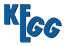REACTION: R12461
Entry
 ```R12461                      Reaction                                ```
Name
protein N6,N6,N6-trimethyl-L-lysine,2-oxoglutarate:oxygen oxidoreductase
Definition
Protein N6,N6,N6-trimethyl-L-lysine + 3 2-Oxoglutarate + 3 Oxygen <=> Protein lysine + 3 Succinate + 3 Formaldehyde + 3 CO2
Equation
C05546 + 3 C00026 + 3 C00007 <=> C02188 + 3 C00042 + 3 C00067 + 3 C00011Comment
three-step reaction (see R12460+R09510+R09511)
Reaction class
 RC01974 C02188_C05546
EnzymeREACTION: R12460
Entry
 ```R12460                      Reaction                                ```
Name
protein N6,N6,N6-trimethyl-L-lysine,2-oxoglutarate:oxygen oxidoreductase
Definition
Protein N6,N6,N6-trimethyl-L-lysine + 2-Oxoglutarate + Oxygen <=> Protein N6,N6-dimethyl-L-lysine + Succinate + Formaldehyde + CO2
EquationComment
the first step of two-step reaction (see R12459, R12460+R09510)
the first step of three-step reaction (see R12461, R12460+R09510+R09511)
Reaction class
 RC00496 C05545_C05546
Enzyme
Other DBs
 RHEA: 61955REACTION: R09510
Entry
 ```R09510                      Reaction                                ```
Name
protein-N6,N6-dimethyl-L-lysine,2-oxoglutarate:oxygen oxidoreductase
Definition
Protein N6,N6-dimethyl-L-lysine + 2-Oxoglutarate + Oxygen <=> Protein N6-methyl-L-lysine + Succinate + Formaldehyde + CO2
EquationComment
the first step of two-step reaction (see R10056, R09510+R09511)
the second step of two-step reaction (see R12459, R12460+R09510)
the second step of three-step reaction (see R12461, R12460+R09510+R09511)
Reaction class
 RC00181 C05544_C05545
 RC00185 C00067_C05545
Enzyme
Other DBs
 RHEA: 61951REACTION: R09511
Entry
 ```R09511                      Reaction                                ```
Name
protein-N6-methyl-L-lysine,2-oxoglutarate:oxygen oxidoreductase
Definition
Protein N6-methyl-L-lysine + 2-Oxoglutarate + Oxygen <=> Protein lysine + Succinate + Formaldehyde + CO2
EquationComment
the second step of two-step reaction (see R10056, R09510+R09511)
the last step of three-step reaction (see R12461, R12460+R09510+R09511)
Reaction class
 RC00060 C02188_C05544
 RC00557 C00067_C05544
Enzyme
Other DBs
 RHEA: 60927